# SCIENCE: Understanding Electricity

THIS IS A QUIZ - IT IS NOT A TEST

It is to help you find correct answers - not to mark you down for incorrect answers!

Please think before answering each question, then skip any you don't understand.

On completing the quiz, you will receive an email (If you have supplied a
valid email address), which will include information to help you (or your
group), to find the correct solution to each incorrect or missing answer.

When you have completed the quiz, please return to the wiki at:

http://wps.flipster.org/doku.php?id=flipsters:electricity:quiz:home

If you have any questions leave a wiki comment, ask classmates or a teacher.

Have fun :)

Adapted from: Science Education Assessment Resources (SEAR) http://cms.curriculum.edu.au/sear/newcms/view_page.asp?page_id=3306

This is a required question
This is a required question
This is a required question
Must contain @
This is a required question
This is a required question

## What is Electricity - The Scientific View: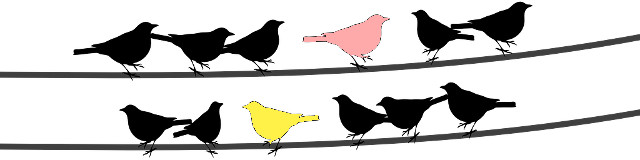## Which sentence(s) do you think best describe(s) what electricity is:

A. Electricity is the electromagnetic field energy sent out by batteries and generators. B. Electricity is the particles: the electrons and protons themselves (the electric charge, not the flow). C. Electricity is the flow of electrons (the flowing motion of the electric charge).
This is a required question
This is a required question
 1 2 3 4 5
This is a required question

## Q1.4 Which diagram(s) best show how a simple electrical circuit works: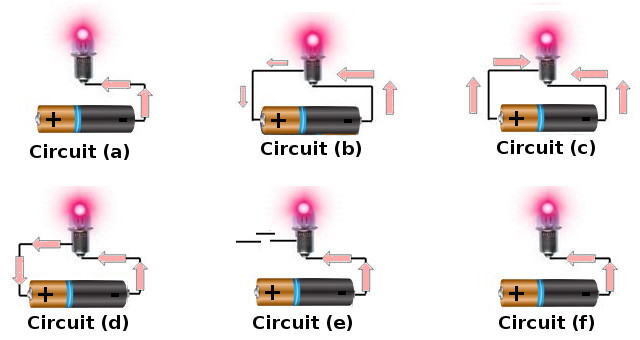This is a required question
This is a required question
 1 2 3 4 5
This is a required question
This is a required question
This is a required question
 1 2 3 4 5
This is a required question
This is a required question
 1 2 3 4 5
This is a required question

## Q2. Big Simple Circuits - Use & Measurement: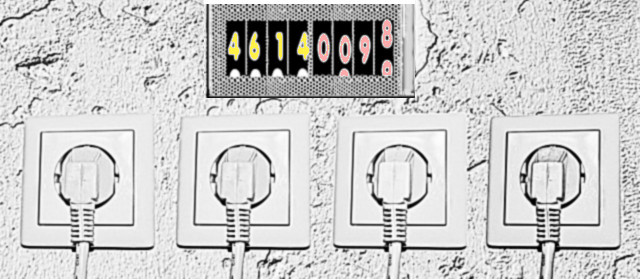This is a required question
 1 2 3 4 5
This is a required question
This is a required question
 1 2 3 4 5
This is a required question

## Q3. Simple Circuits - Speed & Motion:This is a required question
 1 2 3 4 5
This is a required question
This is a required question
 1 2 3 4 5
This is a required question
This is a required question
 1 2 3 4 5
This is a required question

## Q4. Electricity - Path, Work & Flow: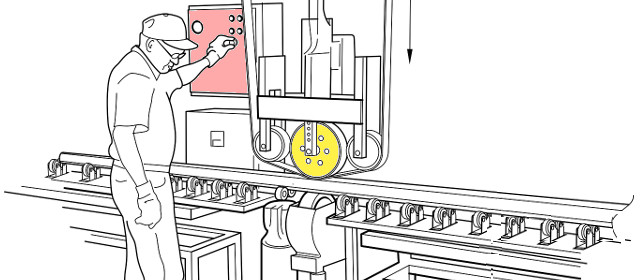This is a required question
 1 2 3 4 5
This is a required question
This is a required question
 1 2 3 4 5
This is a required question

## Q5. Getting There - A Question of Distance vs Speed:This is a required question
This is a required question
 1 2 3 4 5
This is a required question

## If electrons move slowly, why does a light turn on almost instantly when I press a switch that is a long way away:

A. Electrons are arranged like a chain/loop - Tug one end and the opposite end moves almost immediately B. Electrons don't move slowly - they move very fast. C. Speed depends only on thickness of the wire between the lamp & switch D. Electrical energy does not depend on electrons
This is a required question
This is a required question
 1 2 3 4 5
This is a required question

## Q7. Power & Simple Circuits: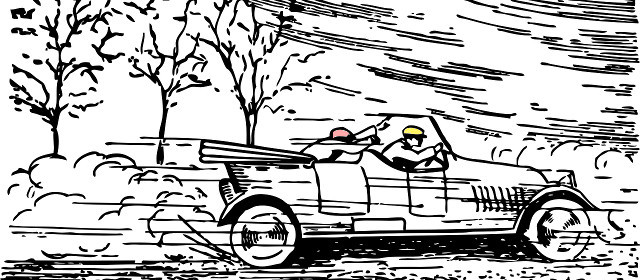This is a required question
 1 2 3 4 5
This is a required question
This is a required question
This is a required question
 1 2 3 4 5
This is a required question## Q8. How energy travels in a simple battery & lamp circuit:

==== DESCRIPTION A ================================== Whenever a battery powers a light bulb, the battery pours out electrical energy into space. That electromagnetic field (EM) energy is then grabbed firmly by the wires and guided by them. The field energy flows parallel to the wires, and eventually it dives into the lightbulb filament. There, it drives the metal's population of movable charges forward, against the resisting force of electrical "friction." Electrons in the metal momentarily speed up before colliding with tungsten atoms. In this way the electrical energy gets converted into thermal energy (heat + light). As a whole, an electric circuit is like a duct for electrical energy, but this duct has no walls. ------------------------------------------------------------------------------------------------------ ==== DESCRIPTION B ================================== Electrical energy flows inside the metal wires. Electrons in the metal wires momentarily speed up before colliding with tungsten atoms. In this way the electrical energy gets converted into thermal energy (heat + light). As a whole, an electric circuit is like a duct for electrical energy. ------------------------------------------------------------------------------------------------------ Please select one of the above options in next question (Q8.1)
This is a required question
This is a required question
 1 2 3 4 5
This is a required question

## How Safe Are Flashlight Batteries:

A. If you had deep cuts & those cuts pressed against the battery terminals, a standard 6V flashlight battery might kill you. B. Standard flashlight batteries are safe & cannot electrocute people. C. Touching a single flashlight battery could electrocute someone with a heart pacemaker.
This is a required question
This is a required question
 1 2 3 4 5
This is a required question